Maths-
General
Easy

Question

# How can you use polynomial identities to factor polynomials and simplify numerical expressions ?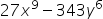Hint:

## The correct answer is: = (3x3 - 7y2)(9x6 + 21x3y2+49y4)

### Step 1 of 2:Factoring a polynomial is the process of decomposing a polynomial into a product of two or more polynomials. This can be done with the help of identities, which would speed up the process and make it simple.Here, the given expression is. This can be written as: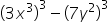where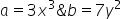.Step 2 of 2:Substitute the values in the expression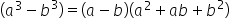for factorization: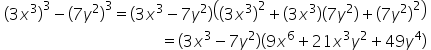We use identities to speed up the process of multiplication and simplification. There are some basic polynomial identities that you need to by heart.#### With Turito Foundation.#### Get an Expert Advice From Turito.